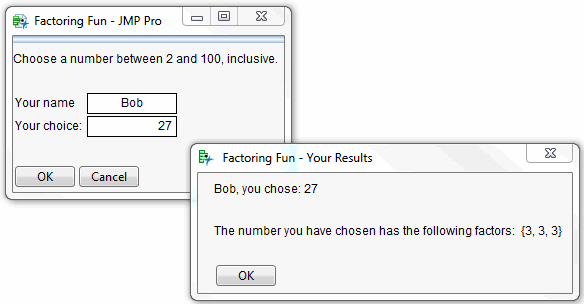Scripting Guide > Common Tasks > Create an Interactive Program
Publication date: 11/29/2021

# Create an Interactive Program

How do you gather numeric input from the users, perform a calculation on that input, and show the results in a new window?

Prime Numbers.jsl asks the user to enter a number and then factors the number or confirms it as a prime number (Figure 17.5). This script is a good example of aligning several types of display boxes, concatenating text, and working with conditional functions.

/* How do you gather numeric input from the users, perform a

calculation on that input, and show the results in a new window?

This script demonstrates how to create an interactive program that

asks the user to enter a number and then factors the number or confirms

it as a prime number. */

// Ask the user to enter a name and number.

`nw = New Window( "Factoring Fun",`
` `
`V List Box(`
`	Text Box( "Choose a number between 2 and 100, inclusive. " ),`
`	Spacer Box( Size( 25, 25 ) )`
`    ),`
`    V List Box(`
`        Lineup Box(`
`            2,`
`            Text Box( "Your name   " ),`
`            uname = Text Edit Box( "< name > ", << Justify Text( Center ) ),`
`            Text Box( "Your choice " ),`
`            uprime = Number Edit Box( 2 )`
`        ),`
`        Spacer Box( Size( 25, 25 ) ),`
`        H List Box(`
`            Button Box( "OK",`

`                username = uname << Get Text;`
`                fromUser0 = uprime << Get;`
` `

// Test input for out of range condition.

`                If( fromUser0 <= 1 | fromUser0 > 100,`

// Send message to user that input value is out of range.

`                    nw2 = New Window( " Factoring Fun: Message for "`
`								|| username,`
`                        <<Modal,`
`                        Text Box(`
`                            "The number you chose, " || Char( fromUser0 ) ||`
`                            " is not between 2 and 100, inclusive.  Please try`
`										again.  "`
`                        ),`
`                        Button Box( "OK" )`
` `
`                    ),`

/* Else the number is within range.

Test for a prime number. If not prime, factor it.

Create a vector which holds the prime numbers

within specified range. */

`                    primes = [2, 3, 5, 7, 11, 13, 17, 19, 23, 29, 31, 37,`
`							41, 43, 47, 53, 59, 61, 67, 71, 73, 79, 83, 89, 97];`

// Count the number of primes in the vector.

`                    p# = N Row( primes );`
` `
`                    isprime = 0; // Set flag.`

// Make a copy of the value for processing.

`							fromuser1 = fromuser0;`
`                    factors = {}; // Initialize list.`
` `

/* Process the value by checking for prime then

factoring if needed. */

`                    While( isPrime == 0,`
` `

// Compare value to vector of prime numbers.

`                        If( Any( fromuser0 == primes ),`

// If found, place value in factor list.

`                            Insert Into( factors, fromUser0 );`
`                            isPrime = 1 // Set condition to exit While loop.`
`                            ;`
`                        ); // End For loop.`
` `
`                        If( isprime == 0,`
`                            For( q = 1, q <= p#, q++,`
`                                If( Mod( fromuser0, primes[q] ) == 0,`
`                                    fromUser0 = fromUser0 / primes[q];`
`                                    Insert Into( factors, primes[q] );`
`                                    q = p# + 1 // End if-then loop.`
`                                    ;`
`                                ); // End If loop.`
`                            ); // End For loop.`
`                        );  // End If/Then loop.`
` `
`                    ); // End while loop.`
` `
`                    cfUser0 = Char( fromUser1 );`
`                    nf = N Items( factors );`
`                    If( nf >= 2,`
`                        fmsg = "The number you have chosen has the following`
`									factors: ",`
`                        fmsg = "The number you have chosen is a prime number: "`
`                    );`

// Show message to user about results.

`                    nw3 = New Window( " Factoring Fun - Your Results",`
`                        <<Modal,`
`                        Text Box( username || ", you chose: " || cfUser0 ),`
`                        Spacer Box( Size( 25, 25 ) ),`
`                        Text Box( fmsg || " " || Char( factors ) ),`
`                        Spacer Box( Size( 25, 25 ) ),`
`                        Button Box( "OK" )`
`                    );`
`                );`
`            ), // End the main OK button script.`

// Close the window and the program.

`            Button Box( "Cancel", nw << Close Window )`
`        )`
`    )`
`);`

Figure 17.5 Factor Numbers Interactively